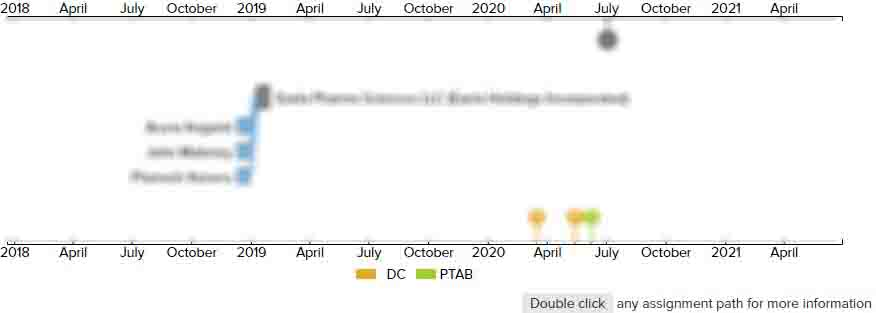# Image processing apparatus, image processing method, and computer program product

• US 20070127837A1
• Filed: 12/06/2006
• Published: 06/07/2007
• Est. Priority Date: 12/06/2005
• Status: Active Grant
##### First Claim
Patent Images

1. An image processing apparatus that receives image information and determines an output gray-scale value of the image information, the image processing apparatus comprising:

• a corrected input-value calculating unit to calculate a corrected input value by assigning a predetermined weight to an error value near a target pixel of the image information and adding the error value to an input gray-scale value;

a threshold selecting unit to select a threshold based on an input gray-scale value of at least one of the target pixel of the image information and a pixel near the target pixel;

an output gray-scale value determining unit to determine an output gray-scale value based on the corrected input value calculated by the corrected input-value calculating unit and the threshold selected by the threshold selecting unit; and

an error calculating unit to calculate a difference between the output gray-scale value determined by the output gray-scale value determining unit and the corrected input value as an error value, and transmit the calculated error value to the corrected input-value calculating unit, wherein when a degree of flexibility is defined as representing a degree of the number of pixels that can take different output values as a result of threshold processing on an input image formed only with a predetermined gray-scale value, the threshold selecting unit selects a threshold from at least two types of threshold tables with same period and different degrees of flexibility based on the input gray-scale value of at least one of the target pixel and the pixel near the target pixel.

• ##### 1 Assignment
Timeline View
Assignment View×
×